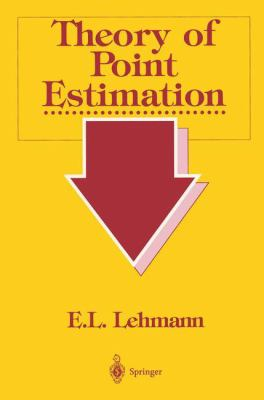## best unbiased estimators

Posted in Books, Kids, pictures, Statistics, University life with tags , , , , , , , , , , , , on January 18, 2018 by xi'anA question that came out on X validated today kept me busy for most of the day! It relates to an earlier question on the best unbiased nature of a maximum likelihood estimator, to which I pointed out the simple case of the Normal variance when the estimate is not unbiased (but improves the mean square error). Here, the question is whether or not the maximum likelihood estimator of a location parameter, when corrected from its bias, is the best unbiased estimator (in the sense of the minimal variance). The question is quite interesting in that it links to the mathematical statistics of the 1950’s, of Charles Stein, Erich Lehmann, Henry Scheffé, and Debabrata Basu. For instance, if there exists a complete sufficient statistic for the problem, then there exists a best unbiased estimator of the location parameter, by virtue of the Lehmann-Scheffé theorem (it is also a consequence of Basu’s theorem). And the existence is pretty limited in that outside the two exponential families with location parameter, there is no other distribution meeting this condition, I believe. However, even if there is no complete sufficient statistic, there may still exist best unbiased estimators, as shown by Bondesson. But Lehmann and Scheffé in their magisterial 1950 Sankhya paper exhibit a counter-example, namely the U(θ-1,θ-1) distribution:since no non-constant function of θ allows for a best unbiased estimator.

Looking in particular at the location parameter of a Cauchy distribution, I realised that the Pitman best equivariant estimator is unbiased as well [for all location problems] and hence dominates the (equivariant) maximum likelihood estimator which is unbiased in this symmetric case. However, as detailed in a nice paper of Gabriela Freue on this problem, I further discovered that there is no uniformly minimal variance estimator and no uniformly minimal variance unbiased estimator! (And that the Pitman estimator enjoys a closed form expression, as opposed to the maximum likelihood estimator.) This sounds a bit paradoxical but simply means that there exists different unbiased estimators which variance functions are not ordered and hence not comparable. Between them and with the variance of the Pitman estimator.

## which parameters are U-estimable?

Posted in Books, Kids, Statistics, University life with tags , , , , , , , on January 13, 2015 by xi'anToday (01/06) was a double epiphany in that I realised that one of my long-time beliefs about unbiased estimators did not hold. Indeed, when checking on Cross Validated, I found this question: For which distributions is there a closed-form unbiased estimator for the standard deviation? And the presentation includes the normal case for which indeed there exists an unbiased estimator of σ, namely$\frac{\Gamma(\{n-1\}/{2})}{\Gamma({n}/{2})}2^{-1/2}\sqrt{\sum_{k=1}^n(x_i-\bar{x})^2}$

which derives directly from the chi-square distribution of the sum of squares divided by σ². When thinking further about it, if a posteriori!, it is now fairly obvious given that σ is a scale parameter. Better, any power of σ can be similarly estimated in a unbiased manner, since$\left\{\sum_{k=1}^n(x_i-\bar{x})^2\right\}^\alpha \propto\sigma^\alpha\,.$

And this property extends to all location-scale models.

So how on Earth was I so convinced that there was no unbiased estimator of σ?! I think it stems from reading too quickly a result in, I think, Lehmann and Casella, result due to Peter Bickel and Erich Lehmann that states that, for a convex family of distributions F, there exists an unbiased estimator of a functional q(F) (for a sample size n large enough) if and only if q(αF+(1-α)G) is a polynomial in 0α1. Because of this, I had this [wrong!] impression that only polynomials of the natural parameters of exponential families can be estimated by unbiased estimators… Note that Bickel’s and Lehmann’s theorem does not apply to the problem here because the collection of Gaussian distributions is not convex (a mixture of Gaussians is not a Gaussian).

This leaves open the question as to which transforms of the parameter(s) are unbiasedly estimable (or U-estimable) for a given parametric family, like the normal N(μ,σ²). I checked in Lehmann’s first edition earlier today and could not find an answer, besides the definition of U-estimability. Not only the question is interesting per se but the answer could come to correct my long-going impression that unbiasedness is a rare event, i.e., that the collection of transforms of the model parameter that are U-estimable is a very small subset of the whole collection of transforms.

## CHANCE: special issue on George Casella’s books

Posted in Books, R, Statistics, University life with tags , , , , , , , on February 10, 2013 by xi'anThe special issue of CHANCE on George Casella’s books has now appeared and it contains both my earlier post on George passing away and  reviews of several of his books, as follows:

Although all of those books have appeared between twenty and five years ago, the reviews are definitely worth reading! (Disclaimer: I am the editor of the Books Review section who contacted friends of George to write the reviews, as well as the co-author of two of those books!) They bring in my (therefore biased) opinion a worthy evaluation of the depths and impacts of those major books, and they also reveal why George was a great teacher, bringing much into the classroom and to his students… (Unless I am confused the whole series of reviews is available to all, and not only to CHANCE subscribers. Thanks, Sam!)